Students can download Maths Chapter 5 Information Processing Ex 5.1 Questions and Answers, Notes, Samacheer Kalvi 6th Maths Guide Pdf helps you to revise the complete Tamilnadu State Board New Syllabus, helps students complete homework assignments and to score high marks in board exams.

## Tamilnadu Samacheer Kalvi 6th Maths Solutions Term 3 Chapter 5 Information Processing Ex 5.1

Question 1.
Study and complete the following pattern.
(i) 1 × 1 = 1
11 × 11 = 121
111 × 111 = 12321
1111 × 1111 = ?
11111 × 11111 = ?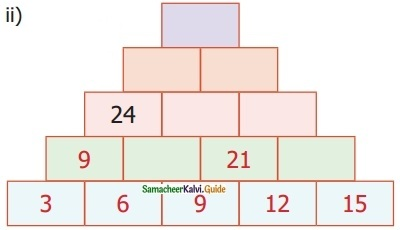Solution:
(i) 1234321, 123454321
(ii) 144, 60, 84, 36, 48, 15, 27Question 2.
Find next three numbers in the following number patterns.
(i) 50, 51, 53, 56, 60……
(ii) 77, 69, 61, 53, ……
(iii) 10, 20, 40, 80,…
(iv) $$\frac{21}{33}$$, $$\frac{321}{444}$$, $$\frac{4321}{555}$$
Solution:

i) The pattern generating these numbers is
50, 50 + 1, 51 + 2, 53 + 3, 56 + 4, 60 + 5, 65 + 6, 71 + 7,
∴ 50, 51, 53, 56, 60, 65, 71, 78, ……
∴ The next three numbers will be 65, 71, 78

ii) The pattern generating these numbers is
77, 77 – 8, 69 – 8, 61 – 8, 53 – 8, 45 – 8, 37 – 8, 29
77, 69, 61, 53, 45, 37, 29, 21,
∴ The next three numbers will be 45, 37, 29.

iii) The pattern generating these numbers is
10, 10 + 10, 20 + 20, 40 + 40, 80 + 80, 160 + 160, 320 + 320,….
10, 20, 40, 80, 160, 320, 640,….
∴ The next three numbers will be 160, 320, 640.

(iv) $$\frac{54321}{66666}$$, $$\frac{654321}{777777}$$, $$\frac{7654321}{8888888}$$

Question 3.
Consider the Fibonacci sequence 1, 1, 2, 3, 5, 8, 13, 21, 34, 55,…. Observe and complete the following table by understanding the number patterns? followed. After filling the table discuss the pattern followed in addition and subtraction, of the numbers of the sequence?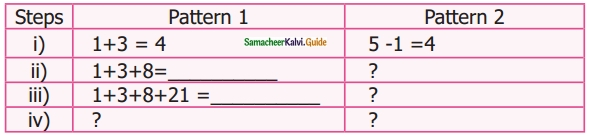Solution:
(i) 12, 13 – 1 = 12
(ii) 33, 34 – 1 = 33
(iii) 1 + 3 + 8 + 21 + 55 = 88, 89 – 1 = 88Question 4.
Complete the following patterns.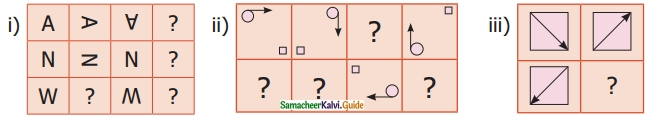Solution: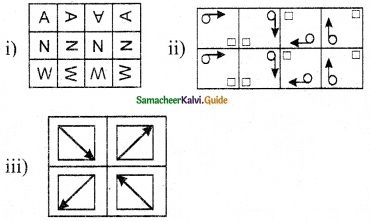Question 5.
Find the HCF of the following pair of numbers by Euclid’s game
(i) 25 and 35
(ii) 36 and 12
(iii) 15 and 29
Solution:
(i) HCF of (25, 35 – 25)
25 = 5 × 5
10 = 2 × 5
HCF of (25, 10) = 5

(ii) HCF of (36, 36 – 12)
36 = 2 × 2 × 3 × 3
24 = 2 × 2 × 2 × 3
HCF of (36, 24) = 2 × 2 × 3 = 12

(iii) HCF of (15, 29 -15)
15 = 3 × 5 × 1
14 = 2 × 7 × 1
HCF of (15, 14) = 1Question 6.
Find HCF of 48 and 28. Also find the HCF of 48 and the number obtained by finding their difference.
Solution:
HCF of 48 and 28
48 = 2 × 2 × 2 × 2 × 3
28 = 2 × 2 × 7
HCF of (48, 28) = 2 × 2 = 4
HCF of (48, 48 – 28)
48 = 2 × 2 × 2 × 2 × 3
20 = 2 × 2 × 5
HCF of (48, 20) = 4

Question 7.
Give instructions to fill in a bank withdrawal form issued in a bank.
Solution:

• The name should be written in capital letters from left to right.
• Write the date of withdrawal on the right top comer of the form.
• Write the amount (in words) to be withdrawn in the space provided.
• Write the amount (in figures) to be withdrawn in the box provided.
• Put your signature at the right bottom above the ‘signature of the depositor’.Question 8.
Arrange the name of your classmates alphabetically.
Solution: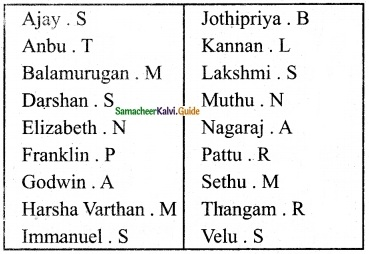Question 9.
Follow and execute the instructions given below.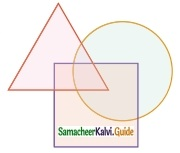(i) Write the number 10 in the place common to the three figures
(ii) Write the number 5 in the place common for square and circle only.
(iii) Write the number 7 in the place common for triangle and circle only.
(iv) Write the number 2 in the place common for triangle and square only.
(v) Write the numbers 12, 14, and 8 only in square, circle, and triangle respectively.
Solution: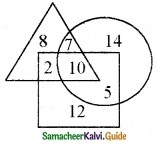Question 10.
Fill in the following information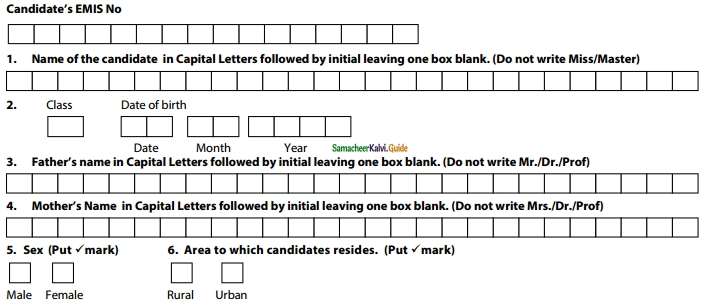Solution: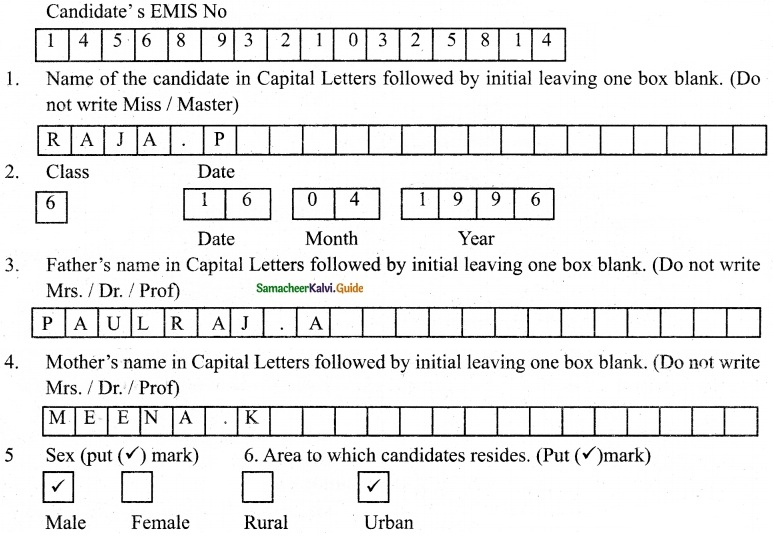Objective Type Questions

Question 11.
The next term in the sequence 15, 17, 20, 22, 25, … is
(a) 28
(b) 29
(c) 27
Hint:
Solution:
(c) 27Question 12.
What will be the 25th letter in the pattern? ABCAABBCCAAABBBCCC,…
(a) B
(b) C
(c) D
(d) A
Solution:
(a) B

Question 13.
The difference between 6th term add 5th term in the Fibonacci sequence is ___.
(a) 6
(b) 8
(c) 5
(d) 3
Solution:
(d) 3Question 14.
The 11th term in the Lucas sequence 1, 3, 4, 7, is
(a) 199
(b) 76
(c) 123
(d) 47
Solution:
(a) 199

Question 15.
If the Highest Common Factor of 26 and 54 is 2, then HCF of 54 and 28 is .
(a) 26
(b) 2
(c) 54
(d) 1
Hint: HCF (54, 28) = HCF (28, 26) = 2
Solution:
(b) 2